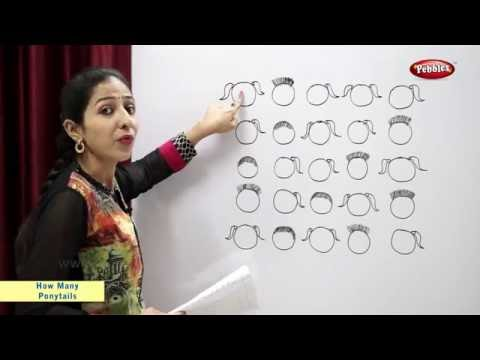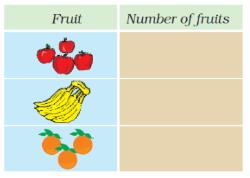Courses

# Test: How Many Ponytails?

## 10 Questions MCQ Test Mathematics for Class 2: NCERT | Test: How Many Ponytails?

Description
This mock test of Test: How Many Ponytails? for Class 2 helps you for every Class 2 entrance exam. This contains 10 Multiple Choice Questions for Class 2 Test: How Many Ponytails? (mcq) to study with solutions a complete question bank. The solved questions answers in this Test: How Many Ponytails? quiz give you a good mix of easy questions and tough questions. Class 2 students definitely take this Test: How Many Ponytails? exercise for a better result in the exam. You can find other Test: How Many Ponytails? extra questions, long questions & short questions for Class 2 on EduRev as well by searching above.
QUESTION: 1

Solution:

12 + 9 = 21

QUESTION: 2

### Susan walks 8 miles. She stops and then walks another 4 miles. How far has she walked in total?

Solution:

8 + 4 is a total of 12

QUESTION: 3

### Last week I watched 6 hours of TV. This week I watched 8 hours. How much TV have I watched altogether?

Solution:

6 + 8 = 14

QUESTION: 4

I have 13 marbles and win 10 more in a game. How many do I have in total?

Solution:

13 + 10 gives a total of 23

QUESTION: 5

I need £2 to go swimming. I have £1.50. How much more do I need?

Solution:

£1.50 + 50p = £2

QUESTION: 6

Joe has 4 pets, Fran has 7 pets, Ben has 2 pets and Sara has 5 pets. What is the total number of pets?

Solution:

4 + 7 + 2 + 5 = 18

QUESTION: 7

Kim is given £9 for her birthday. Jake gets £4 more than this. How much does Jake get?

Solution:

4 more than 9 is 13

QUESTION: 8

An apple costs 20p, an orange costs 10p and a banana costs 5p. How much would 2 apples, 1 orange and 1 banana cost altogether?

Solution:

20p + 20p + 10p + 5p = 55p

QUESTION: 9

A table has 4 legs. How many legs do 4 tables have?

Solution:

4 + 4 + 4 + 4 = 16,
this is the same as 4 x 4

QUESTION: 10

If there are 3 pieces of ribbon that are each 10cm long, how long would they be when added together?

Solution:

10 + 10 + 10 = 30

Track your progress, build streaks, highlight & save important lessons and more!

### Similar Content### Related tests